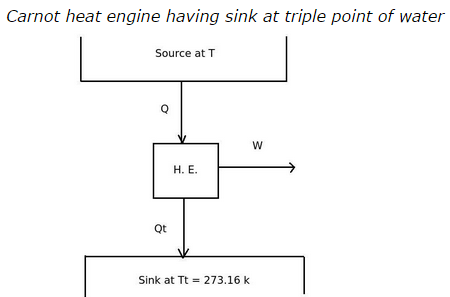# The thermometric property in the Kelvin scale is

## The thermometric property in the Kelvin scale is _______

a. Temperature (T)
b. Work energy (W)
c. Heat energy (Q)
d. all of the above

Correct Answer: c. Heat energy (Q)

Explanation:

Consider the following diagram of heat engine which has sink at triple point of water. The Carnot heat engine takes heat Q from source at temperature T and produce work W and rejects heat Qt at sink which is at triple point of water.Explanation:

According to the definition of Kelvin scale,

Q/Qt = T/ Tt

T = 273.16 (Q/Qt)

From the above equation it is clear that Q that is heat plays a role of thermometric property. The amount of heat supply changes with change is temperature.